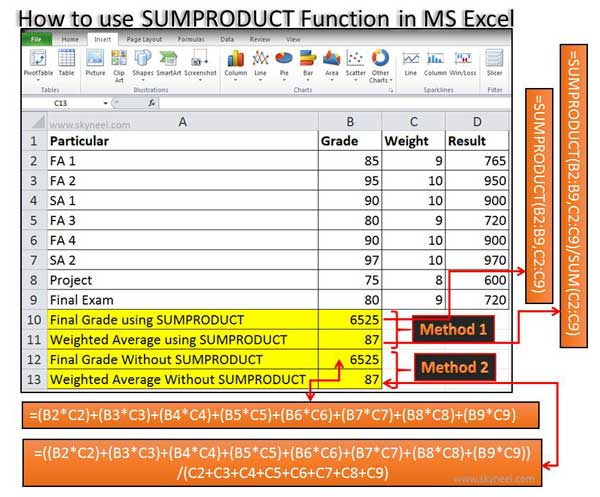# How to use SUMPRODUCT Function in MS Excel

SUMPRODUCT Function in MS Excel is an important math and Trig function.used to return sum of the product of corresponding range or table or array.

All we know very well about the MS Excel. MS Excel is widely used around the world for doing complex calculations in different worksheets, preparing the charts and many more. Functions are the most important part of MS Excel. With the help of functions you can easily get quick result of any complex calculations. Today we discuss about an important SUMPRODUCT Function in MS Excel.

You can use SUMPRODUCT Function to calculate weighted averages of given values.The SUMPRODUCT Function is used to return sum of the product of corresponding range or table or array.

MS Excel is an electronic spreadsheet software which is used to get the solutions of complex calculations, representation numerical data in-term of charts, data analysis, modeling and many more. There are lot of versions of MS Excel. If you saved any worksheet up to MS Excel 2003 then default extension will be *.XLS and if you saved any worksheet to MS Excel 2007 or upper version then it will be *.XLSX.

### SUMPRODUCT Function in MS Excel:

SUMPRODUCT Function in MS Excel is used to calculate weighted averages of given values. The SUMPRODUCT Function is used to return sum of the product of corresponding range or table or array. SUMPRODUCT Function is related to the Math and Trig category.

For eg. If you have student database and now you want to calculate the weighted average with the help of SUMPRODUCT function then you just take few important given steps:

Step 1: Prepare the worksheet as per given below:
Step 2: There are two methods from which you can easily calculate Final Grade of student database:Method 1: If you want to calculate the Final Grade of student database with the help of SUMPRODUCT function then take the cursor on cell B10 and type this formula: =SUMPRODUCT(B2:B9,C2:C9). It will shows you same result 6525.

Method 2: Type the following given formula in Cell B12: =(B2*C2)+(B3*C3)+(B4*C4)+(B5*C5)+(B6*C6)+(B7*C7)+(B8*C8)+(B9*C9) and then press Enter. It will shows you 6525 as a result.

Step 3: If you want to calculate weighted average then again you have to two methods.

Method 1: If you want to calculate the weighted average from SUMPRODUCT function, take the cursor on cell B11 and apply this formula: =SUMPRODUCT(B2:B9,C2:C9)/SUM(C2:C9). After pressing enter you will get 87 as a result.

Method 2: Take the cursor on cell B13 and apply this formula: =((B2*C2)+(B3*C3)+(B4*C4)+(B5*C5)+(B6*C6)+(B7*C7)+(B8*C8)+(B9*C9))/(C2+C3+C4+C5+C6+C7+C8+C9). After pressing enter you will get 87 as a result.

I hope you like this article. After reading this article SUMPRODUCT Function in MS Excel you will be able to easily calculate weighted averages of given values from student database. Kindly share your knowledge with your friends, family members and others. Thanks to all.